Trig Ratios and Cofunction Relationships Trig Ratios SOHCAHTOA

• Slides: 46Trig Ratios and Cofunction Relationships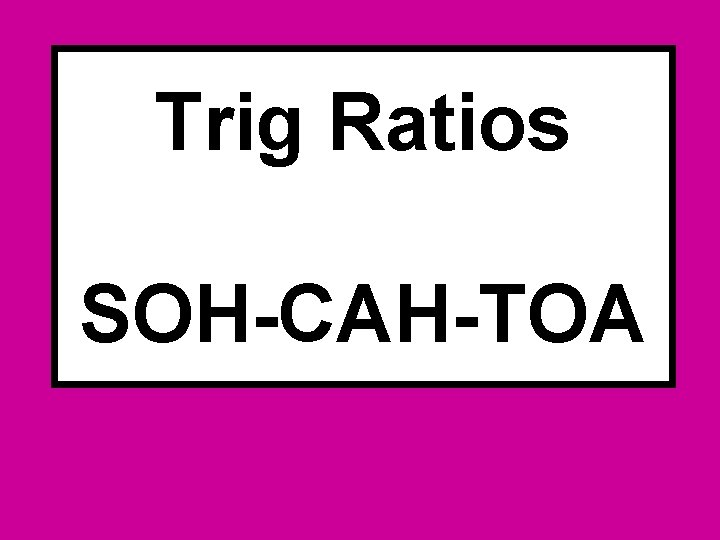Trig Ratios SOH-CAH-TOA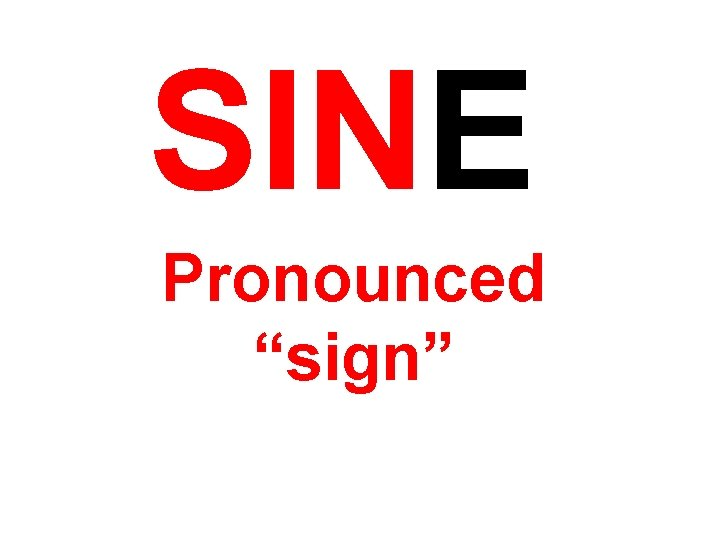SINE Pronounced “sign”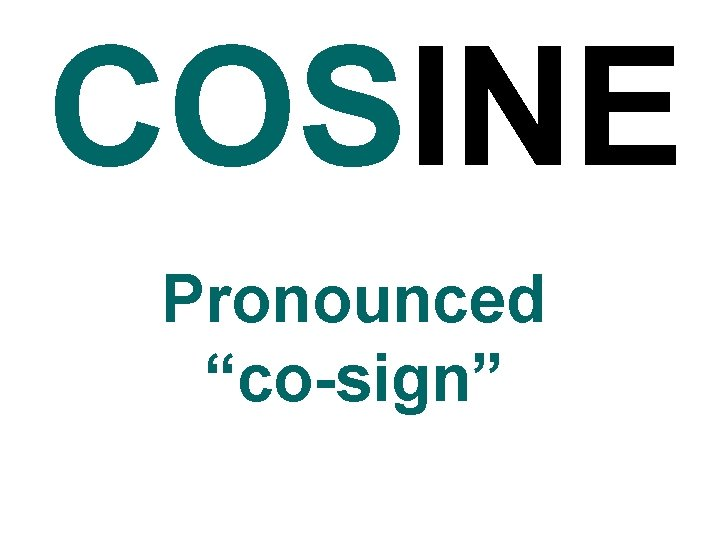COSINE Pronounced “co-sign”TANGENT Pronounced “tan-gent”Greek Letter Pronounced “theta” Represents an unknown angleFinding sin, cos, and tan. Just writing a ratio.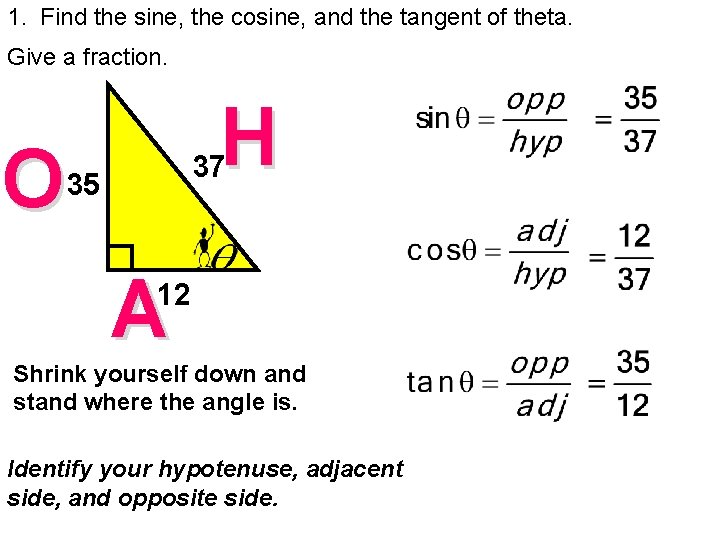1. Find the sine, the cosine, and the tangent of theta. Give a fraction. H O 37 35 A 12 Shrink yourself down and stand where the angle is. Identify your hypotenuse, adjacent side, and opposite side.2. Find the sine, the cosine, and the tangent of theta O 24. 5 8. 2 H 23. 1 A Shrink yourself down and stand where the angle is. Identify your hypotenuse, adjacent side, and opposite side.Sin-Cosine CofunctionThe Sin-Cosine Cofunction7. Sin 28 = ?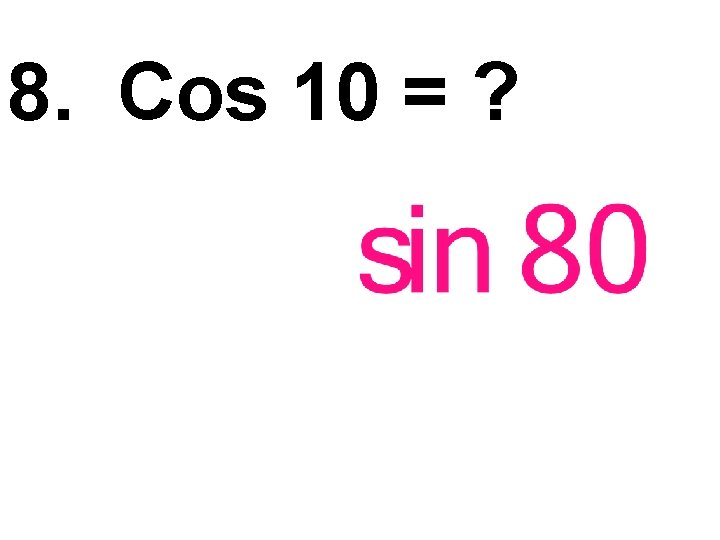8. Cos 10 = ?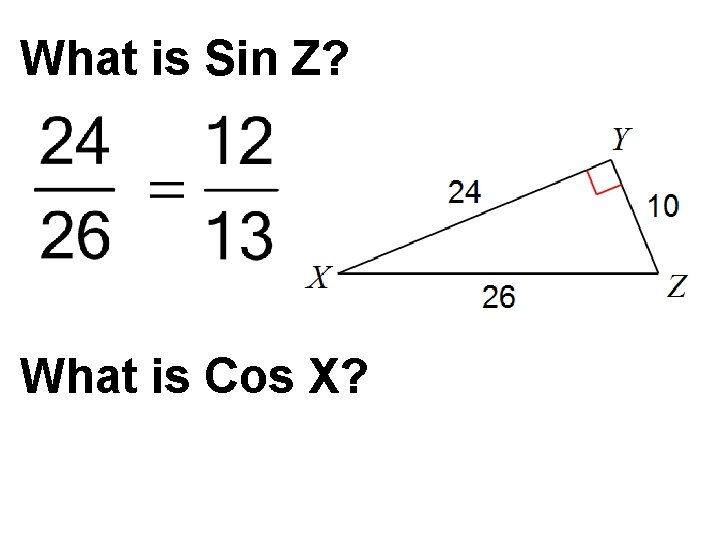What is Sin Z? What is Cos X?What is sin A? What is Cos C?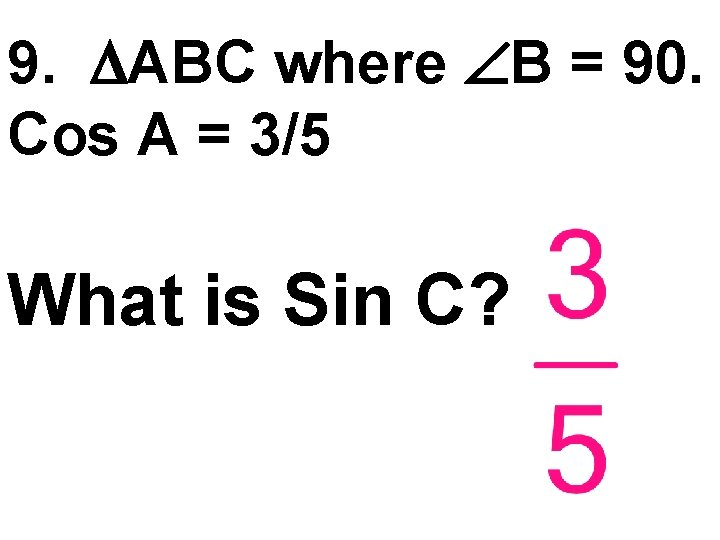9. ABC where B = 90. Cos A = 3/5 What is Sin C?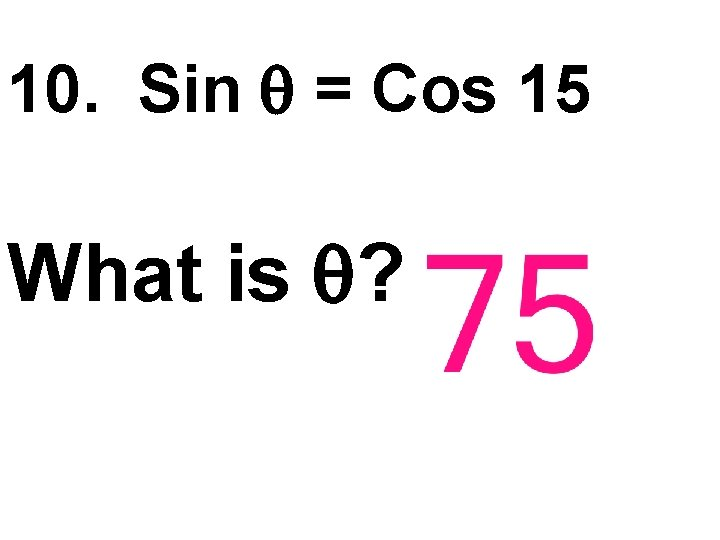10. Sin = Cos 15 What is ?Draw ABC where BAC = 90 and sin B = 3/5 11. What is the length of AB? 4 12. What is tan C? 4/313. Draw stick-man standing where the angle is and mark each given side. Then tell which trig ratio you have. sin O HC 5 A 2 M 4. If C = 20º, then cos C is equal to: A. sin 70 B. cos 70 C. tan 70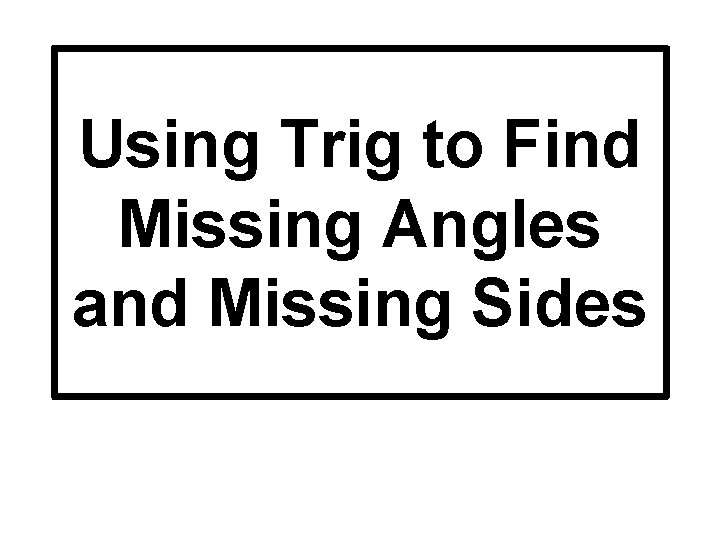Using Trig to Find Missing Angles and Missing SidesFinding a missing angle. (Figuring out which ratio to use and an inverse trig button. )Ex: 1 Figure out which ratio to use. Find x. Round to the nearest tenth. O 20 m A 40 m x Shrink yourself down and stand where the angle is. Identify the given sides as H, O, or A. What trig ratio is this?Ex: 2 Figure out which ratio to use. Find x. Round to the nearest tenth. O 15 m H 50 m x Shrink yourself down and stand where the angle is. Identify the given sides as H, O, or A. What trig ratio is this?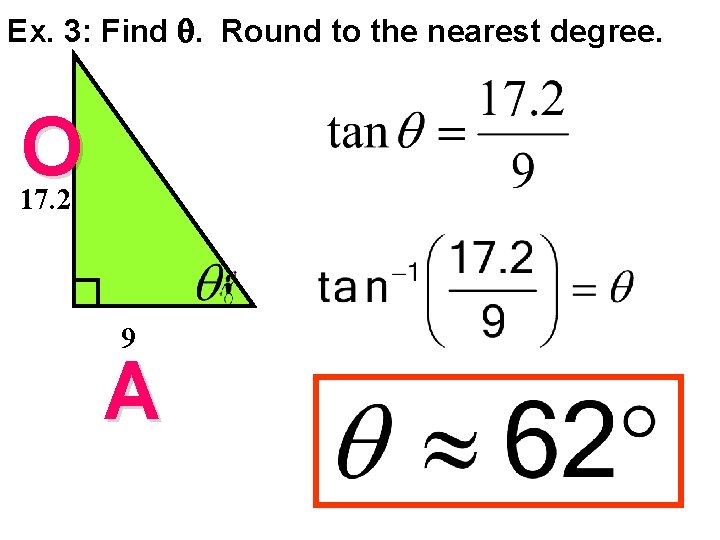Ex. 3: Find . Round to the nearest degree. O 17. 2 9 A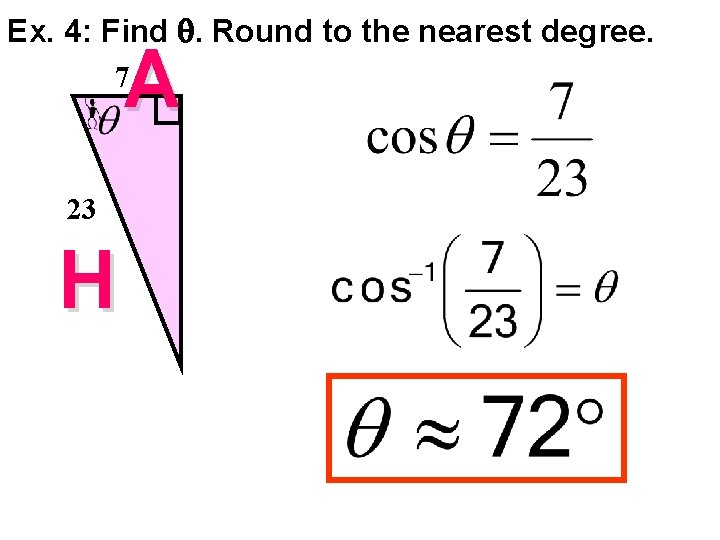Ex. 4: Find . Round to the nearest degree. A 7 23 H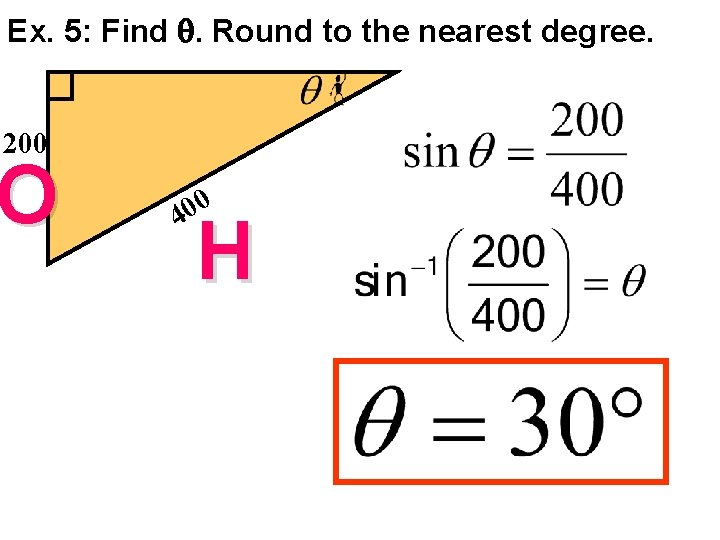Ex. 5: Find . Round to the nearest degree. 200 O 0 0 4 H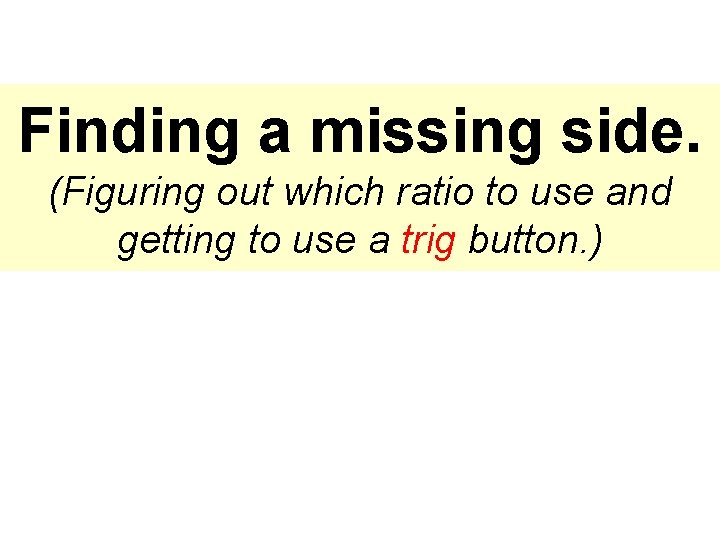Finding a missing side. (Figuring out which ratio to use and getting to use a trig button. )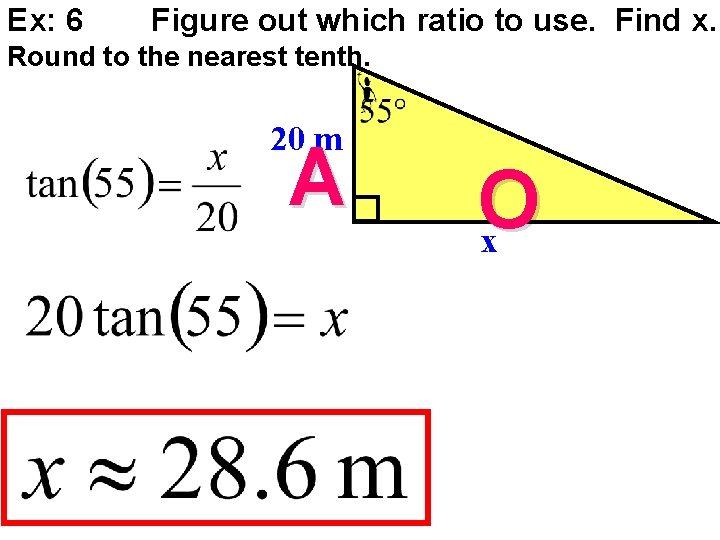Ex: 6 Figure out which ratio to use. Find x. Round to the nearest tenth. 20 m A O xEx: 7 Find the missing side. Round to the nearest tenth. x A O 80 ft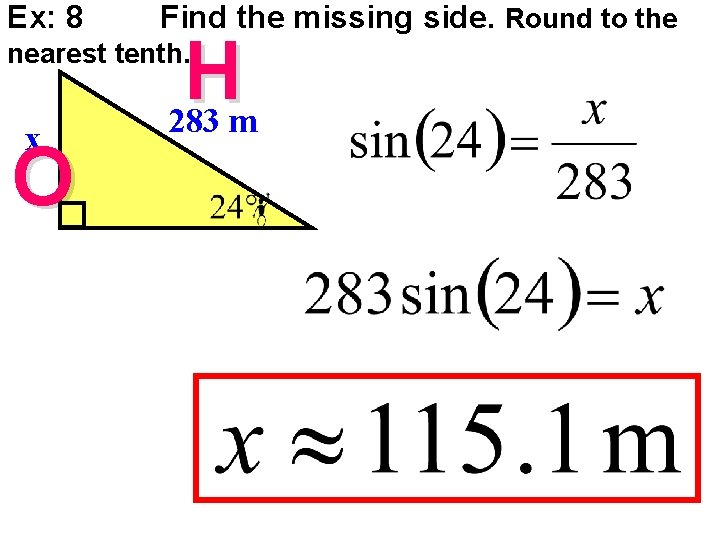Ex: 8 Find the missing side. Round to the H 283 m nearest tenth. x O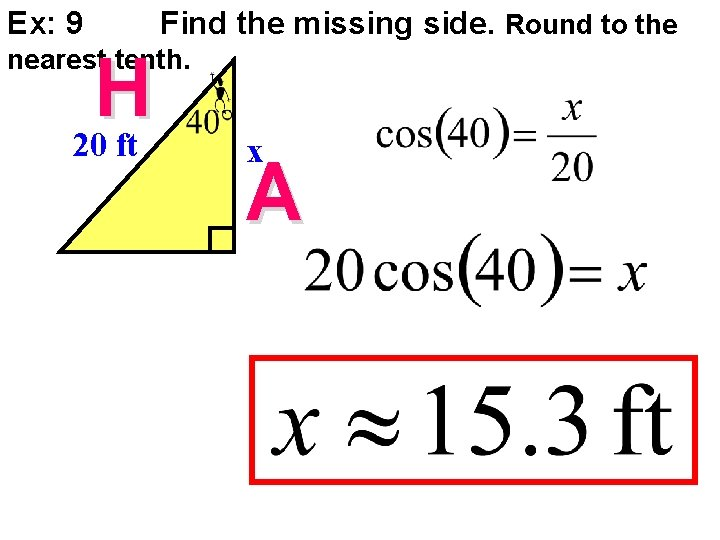Ex: 9 Find the missing side. Round to the nearest tenth. H 20 ft x A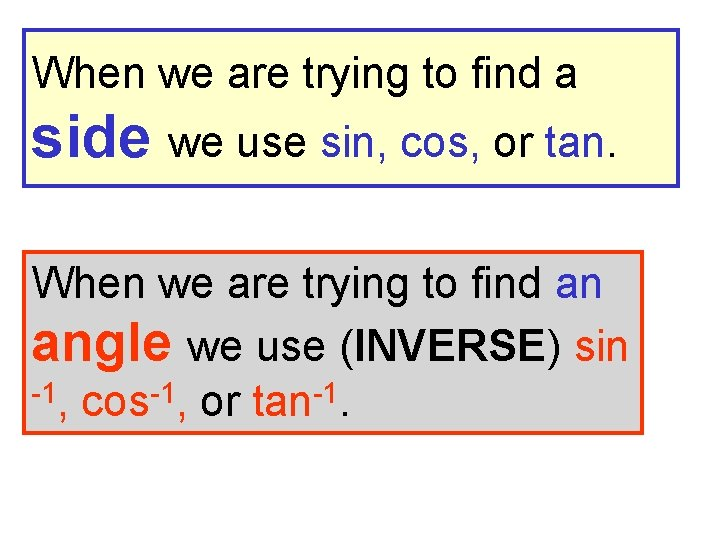When we are trying to find a side we use sin, cos, or tan. When we are trying to find an angle we use (INVERSE) sin -1, cos-1, or tan-1.Trig Application Problems MM 2 G 2 c: Solve application problems using the trigonometric ratios.Depression and Elevation angle of depression horizontal t line gh i s f o angle of elevation horizontal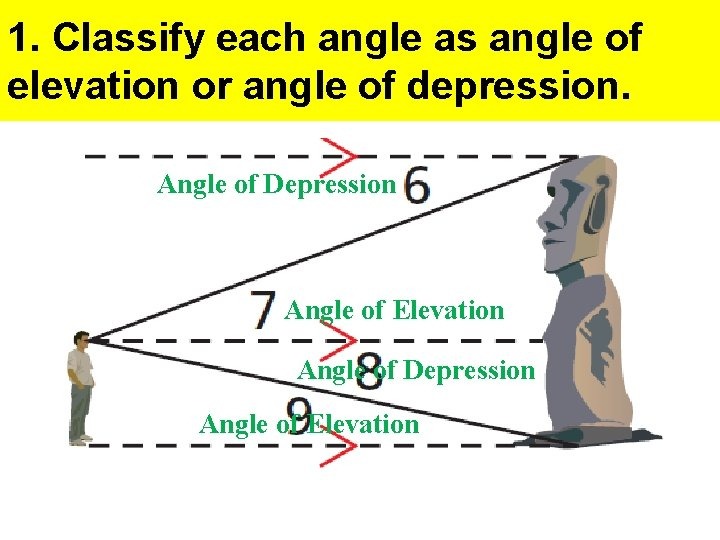1. Classify each angle as angle of elevation or angle of depression. Angle of Depression Angle of ElevationExample 2 • Over 2 miles (horizontal), a road rises 300 feet (vertical). What is the angle of elevation to the nearest degree? 5280 feet – 1 mile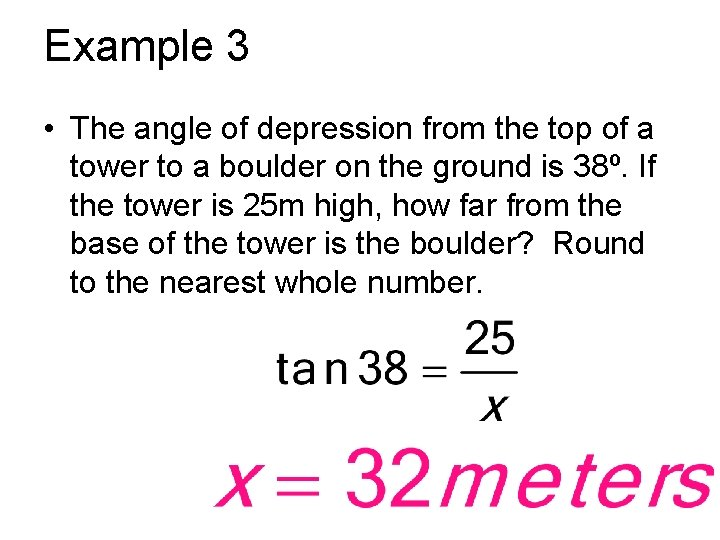Example 3 • The angle of depression from the top of a tower to a boulder on the ground is 38º. If the tower is 25 m high, how far from the base of the tower is the boulder? Round to the nearest whole number.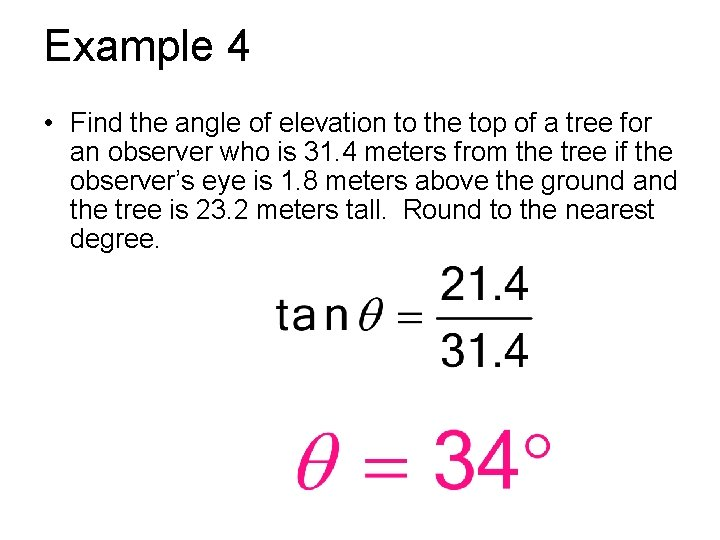Example 4 • Find the angle of elevation to the top of a tree for an observer who is 31. 4 meters from the tree if the observer’s eye is 1. 8 meters above the ground and the tree is 23. 2 meters tall. Round to the nearest degree.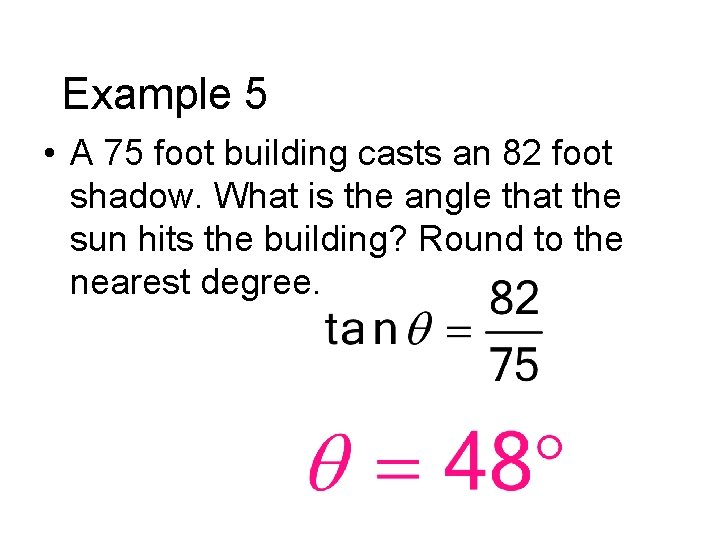Example 5 • A 75 foot building casts an 82 foot shadow. What is the angle that the sun hits the building? Round to the nearest degree.Example 6 • A boat is sailing and spots a shipwreck 650 feet below the water. A diver jumps from the boat and swims 935 feet to reach the wreck. What is the angle of depression from the boat to the shipwreck, to the nearest degree?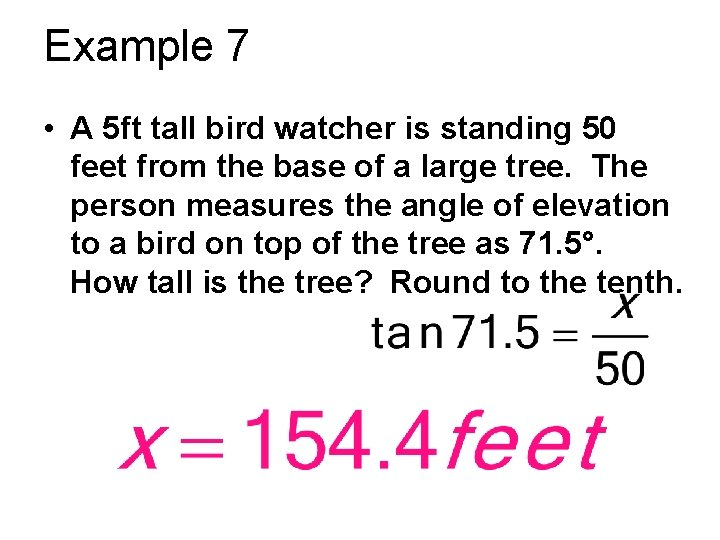Example 7 • A 5 ft tall bird watcher is standing 50 feet from the base of a large tree. The person measures the angle of elevation to a bird on top of the tree as 71. 5°. How tall is the tree? Round to the tenth.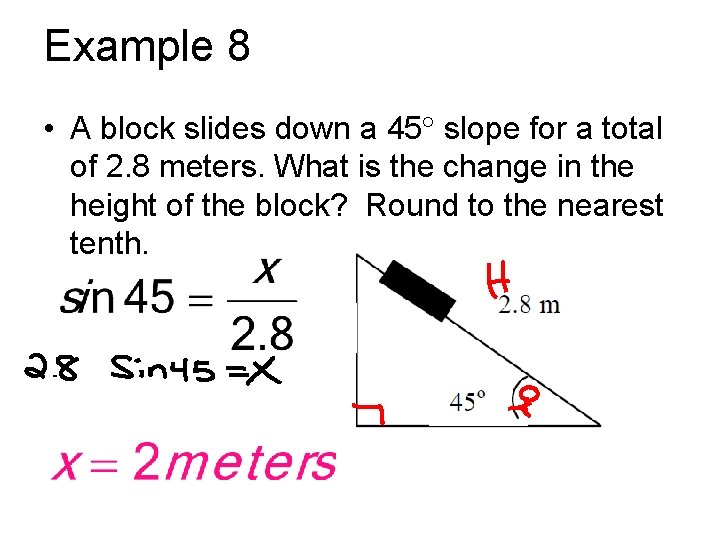Example 8 • A block slides down a 45 slope for a total of 2. 8 meters. What is the change in the height of the block? Round to the nearest tenth.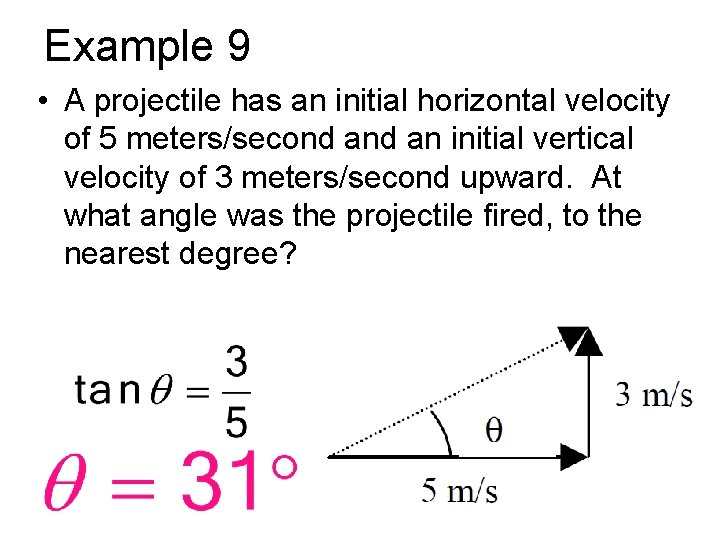Example 9 • A projectile has an initial horizontal velocity of 5 meters/second an initial vertical velocity of 3 meters/second upward. At what angle was the projectile fired, to the nearest degree?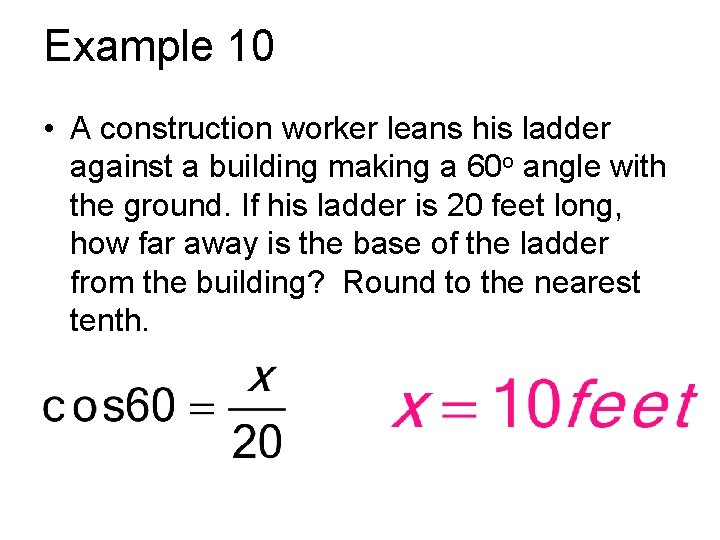Example 10 • A construction worker leans his ladder against a building making a 60 o angle with the ground. If his ladder is 20 feet long, how far away is the base of the ladder from the building? Round to the nearest tenth.